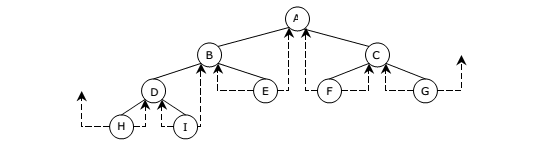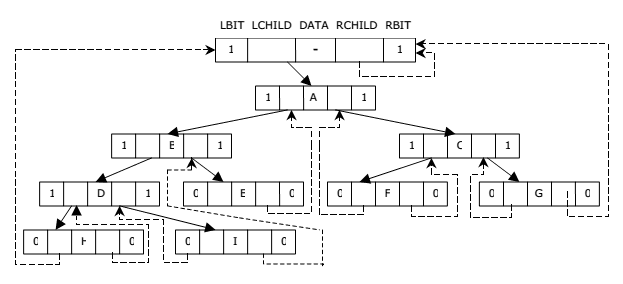# Threaded Binary Tree A+ A  A-

The linked representation of any binary tree has more null links than actual pointers. If there are 2n total links, there are n+1 null links. A clever way to make use of these null links has been devised by A.J. Perlis and C. Thornton.

Their idea is to replace the null links by pointers called Threads to other nodes in the tree.

If the RCHILD(p) is normally equal to zero, we will replace it by a pointer to the node which would be printed after P when traversing the tree in inorder.

A null LCHILD link at node P is replaced by a pointer to the node which immediately precedes node P in inorder. For example, Let us consider the tree:The Threaded Tree corresponding to the above tree is:The tree has 9 nodes and 10 null links which have been replaced by Threads. If we traverse T in inorder the nodes will be visited in the order H D I B E A F C G.

For example, node ‘E’ has a predecessor Thread which points to ‘B’ and a successor Thread which points to ‘A’. In memory representation Threads and normal pointers are distinguished between as by adding two extra one bit fields LBIT and RBIT.

LBIT(P) = 1 if LCHILD(P) is a normal pointer

LBIT(P) = 0 if LCHILD(P) is a Thread

RBIT(P) = 1 if RCHILD(P) is a normal pointer

RBIT(P) = 0 if RCHILD(P) is a Thread

In the above figure two threads have been left dangling in LCHILD(H) and RCHILD(G). In order to have no loose Threads we will assume a head node for all threaded binary trees. The Complete memory representation for the tree is as follows. The tree T is the left sub-tree of the head node.